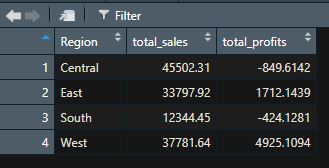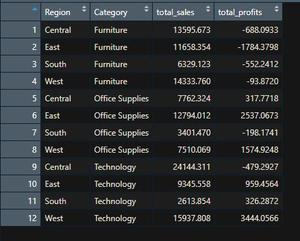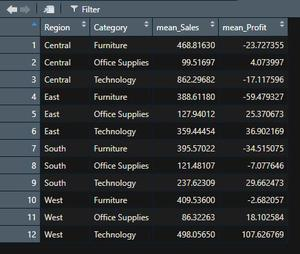Open In App

# Group by function in R using Dplyr

Group_by() function belongs to the dplyr package in the R programming language, which groups the data frames. Group_by() function alone will not give any output. It should be followed by summarise() function with an appropriate action to perform. It works similar to GROUP BY in SQL and pivot table in excel.

Syntax:

group_by(col,…)

Syntax:

group_by(col,..) %>% summarise(action)

The dataset in use:

Sample_Superstore

## Group_by() on a single column

This is the simplest way by which a column can be grouped, just pass the name of the column to be grouped in the group_by() function and the action to be performed on this grouped column in summarise() function.

Example: Grouping single column by group_by()

## R

 `library``(dplyr)` `df = ``read.csv``(``"Sample_Superstore.csv"``)` `df_grp_region = df %>% ``group_by``(Region)  %>%``                    ``summarise``(total_sales = ``sum``(Sales),``                              ``total_profits = ``sum``(Profit),``                              ``.groups = ``'drop'``)` `View``(df_grp_region)`

Output:## Group_by() on multiple columns

Group_by() function can also be performed on two or more columns, the column names need to be in the correct order. The grouping will occur according to the first column name in the group_by function and then the grouping will be done according to the second column.

Example: Grouping multiple columns

## R

 `library``(dplyr)` `df = ``read.csv``(``"Sample_Superstore.csv"``)` `df_grp_reg_cat = df %>% ``group_by``(Region, Category) %>%``                   ``summarise``(total_Sales = ``sum``(Sales),``                             ``total_Profit = ``sum``(Profit),``                             ``.groups = ``'drop'``)` `View``(df_grp_reg_cat)`

Output:We can also calculate mean, count, minimum or maximum by replacing the sum in the summarise or aggregate function. For example, we will find mean sales and profits for the same group_by example above.

Example:

## R

 `library``(dplyr)` `df = ``read.csv``(``"Sample_Superstore.csv"``)` `df_grp_reg_cat = df %>% ``group_by``(Region, Category) %>%``                   ``summarise``(mean_Sales = ``mean``(Sales),``                             ``mean_Profit = ``mean``(Profit),``                             ``.groups = ``'drop'``)` `View``(df_grp_reg_cat)`

Output: